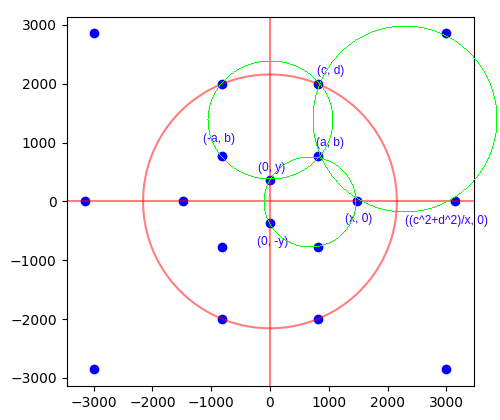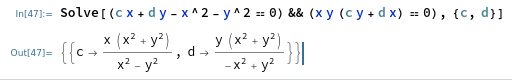# Infinitely many rational dodecahedra

Thomas Blok and David Madore have recently made significant progress on the problem of finding rational dodecahedra inscribed in the unit sphere, culminating in an infinite parametric family of solutions.

In particular, Thomas began with the constrained version of the problem that I used to find the first solution: namely where the dodecahedron has an order-8 symmetry group generated by reflections in three orthogonal planes. After stereographic projection, we are left with the following picture:These solutions are parametrised by six positive rational variables, {a, b, c, d, x, y}, as shown in the image above. Thomas made the observation that if we fix {c, d, x, y} and draw the three green circles, then if they intersect at a common point (a, b), that common point must necessarily be rational.

Consequently, the problem becomes much simpler:

Find positive rationals (x, y, c, d) with y < x < sqrt(c² + d²) such that the circumcircles of the three triangles:

• {(0, −y), (0, y), (x, 0)};
• {(0, y), (−c, d), (c, d)};
• {(x, 0), (c, d), ((c² + d²)/x, 0)};

all mutually intersect at a single point.

David Madore responded to Blok’s MathOverflow question and found a degree-18 polynomial equation in 18 variables expressing the cointersection of three circles defined by 9 points. Madore’s polynomial has 27 873 486 terms, so it is rather unwieldy to manipulate.

With the additional constraint that the 9 points form a 3-by-3 symmetric matrix (thus there are only 12 distinct variables), as is the case for the dodecahedron problem, Madore discovered that the degree-18 polynomial has only 1 980 078 terms and factors as a product of three ‘boring’ degree-4 factors (corresponding to degenerate cases where some points coincide) and an ‘interesting’ degree-6 factor with 720 terms.

Blok substituted the coordinates from the dodecahedron problem into the degree-6 polynomial, obtaining a degree-8 polynomial equation in the variables {x, y, c, d}. Blok observed that it is again reducible, with ‘boring’ factors$cy(c^2 + d^2 - x^2)$ and a degree-4 ‘interesting’ factor that can be written as:$xy(cy + dx) + (c^2 + d^2)(cx + dy - x^2 - y^2) = 0$

This is homogeneous, because global scale factors do not affect whether a solution is valid or not. As such, fixing one variable (such as setting y = 1) merely fixes the global scale factor. On the other hand, fixing a second variable (such as setting x = 4) does remove a degree of freedom from the solution space. When fixing x = 4 and y = 1, as suggested, we obtain the following equation in the remaining variables:$c^2 d+4 c^3-17 c^2+4 c d^2+4 c+d^3-17 d^2+16 d = 0$

Observe that this is a cubic equation rather than a quartic equation. (The same is true if we simultaneously fix c and d instead of x and y.) As such, it has an elliptic curve group law, and we can therefore construct new solutions from existing solutions:The uppermost point here is the rational solution that was found by computer search. The remaining points in the diagram are generated from that point together with the point at infinity (which, unlike in Weierstrass curves, appears not to be a neutral element). Proceeding in this manner, we can produce infinitely many solutions with fixed x and y provided that we have at least one solution that is not a torsion point on the elliptic curve.

### The point at infinity

We observed that the point at infinity does not appear to be a neutral element, so perhaps we can use that to generate solutions without needing an initial solution. In particular, this should work with any choices of x and y.

Note that the equation has two terms:$xy(cy + dx) + (c^2 + d^2)(cx + dy - x^2 - y^2) = 0$

We can divide both terms by$c^2 + d^2$ to obtain an equivalent equation:$\frac{xy(cy + dx)}{(c^2 + d^2)} + (cx + dy - x^2 - y^2) = 0$

The first term tends to zero as we approach infinity; the remaining terms are a linear equation in c and d. Consequently, the equation after discarding the first term…$cx + dy - x^2 - y^2 = 0$

…is precisely the equation of the asymptote λ of the elliptic curve! Intersecting this with the line at infinity gives the point at infinity ∞ on the elliptic curve, at which the line λ is tangent. However, λ must also intersect the curve at another point, P, satisfying P + ∞ + ∞ = 0 (in the elliptic curve group law).

The point P is precisely the point where both terms in the elliptic curve equation vanish:This is exciting, because it gives us a rational point on the correct component (the unbounded one) of the elliptic curve. Unfortunately, one of the two coordinates is negative, so this is not a valid solution to the dodecahedron problem (if you apply stereographic projection then some of the dodecahedron’s pentagonal faces intersect each other).

However, we can repeat the strategy of drawing a tangent line at this point and letting it reintersect our elliptic curve. This gives the following solution:$c = \dfrac{x \left(x^2+y^2\right) \left(2 x^2 y^2+x^4+5 y^4\right) \left(-3 x^6 y^2-2 x^4 y^4-3 x^2 y^6+x^8-y^8\right)}{(x-y) (x+y) \left(11 x^{10} y^2+11 x^8 y^4+18 x^6 y^6+11 x^4 y^8+11 x^2 y^{10}+x^{12}+y^{12}\right)}$$d = \dfrac{y \left(x^2+y^2\right) \left(2 x^2 y^2+5 x^4+y^4\right) \left(3 x^6 y^2+2 x^4 y^4+3 x^2 y^6+x^8-y^8\right)}{(x-y) (x+y) \left(11 x^{10} y^2+11 x^8 y^4+18 x^6 y^6+11 x^4 y^8+11 x^2 y^{10}+x^{12}+y^{12}\right)}$

which is positive when x ≥ 1.9397y and has a simple expression for the norm:$c^2 + d^2 = \dfrac{(x^2 + y^2)^3}{(x^2 - y^2)^2}$

which, in particular, implies that the points (x, 0) and (0, y) are definitely inside the circle of inversion passing through (c, d). Consequently, not only is this an algebraic solution to the problem, but also it yields a valid geometric solution. The intersection points are given by more complicated rational functions of x and y:$a = \frac{x (x-y) (x+y) \left(2 x^2 y^2+x^4+5 y^4\right) \left(x^6 y^2+4 x^4 y^4+x^2 y^6+x^8+y^8\right) \left(11 x^{10} y^2+11 x^8 y^4+18 x^6 y^6+11 x^4 y^8+11 x^2 y^{10}+x^{12}+y^{12}\right)}{38 x^{24} y^2+111 x^{22} y^4+310 x^{20} y^6+459 x^{18} y^8+792 x^{16} y^{10}+682 x^{14} y^{12}+804 x^{12} y^{14}+379 x^{10} y^{16}+414 x^8 y^{18}+47 x^6 y^{20}+70 x^4 y^{22}-15 x^2 y^{24}+x^{26}+4 y^{26}}$$b = \frac{y \left(2 x^2 y^2+5 x^4+y^4\right) \left(4 x^4 y^2+x^2 y^4+x^6+2 y^6\right) \left(3 x^6 y^2+2 x^4 y^4+3 x^2 y^6+x^8-y^8\right) \left(x^6 y^2+2 x^4 y^4+x^2 y^6+x^8+3 y^8\right)}{38 x^{24} y^2+111 x^{22} y^4+310 x^{20} y^6+459 x^{18} y^8+792 x^{16} y^{10}+682 x^{14} y^{12}+804 x^{12} y^{14}+379 x^{10} y^{16}+414 x^8 y^{18}+47 x^6 y^{20}+70 x^4 y^{22}-15 x^2 y^{24}+x^{26}+4 y^{26}}$

Excitingly, when x and y are the two legs of a Pythagorean triple, the radius of the circle of inversion is itself rational, so we can scale down so that the circle of inversion is the unit circle. That means that, after stereographic projection, the dodecahedron is symmetric under reflection in the three coordinate planes.

Taking the (5, 12, 13) Pythagorean triple, the resulting dodecahedron has the following 20 vertices:

• 4 vertices of the form (0, ±1307215, ±2236392)/2590417;
• 4 vertices of the form (±6274632, 0, ±2787625)/6865993;
• 4 vertices of the form (±64472300514372, ±382579158329275, 0)/387973568586253;
• 8 vertices of the form (±386997531010983823086099699996473688, ±553410551662400041405804573591217100, ±568341850739218062368814809404334875) /882634005124184502065650762315319437;

all of which are rational points on the unit sphere.Since we can generate Pythagorean triples parametrically, this gives us an infinite parametric family of rational dodecahedra in the unit sphere which are symmetric under reflections in the three coordinate axes.

Is it possible to find rational dodecahedra arbitrarily close to a regular dodecahedron? It seems that this should be the case, because (torsion points notwithstanding) each solution should generate a dense subset of the points on the correct component of the elliptic curve.

This entry was posted in Uncategorized. Bookmark the permalink.

### 6 Responses to Infinitely many rational dodecahedra

1.tomtom2357 says:

Awesome, that is exactly what I was thinking in terms of a parametrized family of solutions. It’s fascinating that you can get rational dodecahedra where the side lengths are so different. I have another question. Is it possible that for some rational x and y (or c and d), the cubic curve becomes singular? That would make the parametric equations for the curve far simpler.

2.Simon Tatham says:

Rational dodecahedra arbitrarily close to a regular dodecahedron:

I haven’t actually tried this, partly because I’m not 100% sure I’ve understood every step in this derivation (and have work to do), and also because the huge algebraic formulae show up in my browser as images, making them rather hard to copy-paste into any calculating system.

But if I’ve understood all of this correctly, we only have to find a Pythagorean triple, and then generate a,b,c,d in terms of that by formulae that describe continuous functions, and then scale down to the unit sphere? And so, if we can find a sequence of triples such that the ratios y/x converge to the ratio of the irrational values obtained from the stereographic projection of the regular dodecahedron, that ought to give tuples (x,y,a,b,c,d) all of whose elements converge to the full tuple corresponding to the regular dodecahedron?

If so, then it’s easy to generate Pythagorean triples (x,y,z) with x/y converging to a value of your choice, because really a Pythagorean triple is a square Gaussian integer, and squaring doubles the argument. So pick any old sequence of Gaussian integers whose arguments converge to half the argument you’re aiming for, e.g. taking real and imaginary parts to be the denominator and numerator of the continued fraction convergents to tan(½ arctan y/x), and square them all to get a sequence of Pythagorean triples converging similarly to what you were aiming for.

•apgoucher says:

The space of dodecahedra (inscribed in the unit sphere and invariant under reflections in the three coordinate axes) is a two-dimensional surface, with the regular dodecahedron being an (irrational) point on that surface. The infinite family I parametrised is a dense subset of some curve drawn on that surface, but that curve probably doesn’t contain the point corresponding to the regular dodecahedron.

3.Someperson says:

By fixing c and q instead, the curve has (c,0) as a rational point, and the other intersection of the tangent at (c,0) gives the rational point (x,y)=(c+d^2/c,d(c^2+d^2)/(2c^2+d^2)). This yields a simpler parameteric formula.

•Someperson says:

Unfortunately, it seems that this is x is always greater than sqrt(c^2+d^2)

•Someperson says:

Starting from the point (0,d) and using the fact that lines of slope 0, infinity, and -d/c all intersect the curve at infinity, I found a simple, geometrically valid solution of (x,y)=(2d^2(c^2+d^2)/(c(c^2+3d^2)),d(d^2-c^2)/(c^2+d^2)). At least, it’s valid in a region of positive area that includes (c,d)=(.6,.8).